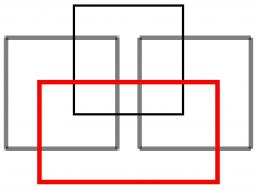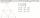# Rectangle

The perimeter of the rectangle is 22 cm and content area 30 cm2. Determine its dimensions, if the length of the sides of the rectangle in centimeters is expressed by integers.

a =  5 cm
b =  6 cm

### Step-by-step explanation:

a=1; b=11-a=10; S = ab=10
a=2; b=11-a=9; S = ab=18
a=3; b=11-a=8; S = ab=24
a=4; b=11-a=7; S = ab=28
a=5; b=11-a=6; S = ab=30 <<<<<< OK
a=6; b=11-a=5; S = ab=30 <<<<<< OK
a=7; b=11-a=4; S = ab=28
a=8; b=11-a=3; S = ab=24
a=9; b=11-a=2; S = ab=18
a=10; b=11-a=1; S = ab=10

The equations have the following integer solutions:
2*(a+b)=22
ab = 30

Number of solutions found: 2
##### a1=5, b1=6a2=6, b2=5

Calculated by our Diofant problems and integer equations.Did you find an error or inaccuracy? Feel free to write us. Thank you!Tips to related online calculators
Looking for help with calculating roots of a quadratic equation?
Do you solve Diofant problems and looking for a calculator of Diofant integer equations?

#### You need to know the following knowledge to solve this word math problem:

We encourage you to watch this tutorial video on this math problem:

## Related math problems and questions:

• Rectangle diagonalsIt is given a rectangle with an area of 24 cm2 a circumference of 20 cm. The length of one side is 2 cm larger than the length of the second side. Calculate the length of the diagonal. Length and width are yet expressed in natural numbers.
• Perimeter and legsDetermine the perimeter of a right triangle if the length of one leg is 75% length of the second leg and its content area is 24 cm2.
• RectangleThe width of the rectangle is 65% of its length. Perimeter of the rectangle is 132 cm. Determine the dimensions of the rectangle.
• SquareSuppose the square's sides' length decreases by a 25% decrease in the content area of 28 cm2. Determine the side length of the original square.
• Do you solve this?Determine area S of rectangle and length of its sides if its perimeter is 102 cm.
• Two rectanglesI cut out two rectangles with 54 cm², 90 cm². Their sides are expressed in whole centimeters. If I put these rectangles together I get a rectangle with an area of 144 cm2. What dimensions can this large rectangle have? Write all options. Explain your calc
• Rectangles - sidesOne side of the rectangle is 10 cm longer than second. Shortens longer side by 6 cm and extend shorter by 14 cm increases the area of the rectangle by 130 cm2. What are the dimensions of the original rectangle?
• Rectangle - sidesWhat is the perimeter of a rectangle with area 266 cm2 if length of the shorter side is 5 cm shorter than the length of the longer side?
• Percentage and rectangleAbout what percentage increases perimeter and area of a rectangle if both the sides 12 cm and 10 cm long we increase by 20%?
• TilesFrom how many tiles 20 cm by 30 cm, we can build a square of maximum dimensions if we have maximum 881 tiles.
• Rhombus and inscribed circleIt is given a rhombus with side a = 6 cm and the radius of the inscribed circle r = 2 cm. Calculate the length of its two diagonals.
• The rectangleDetermine the area of the rectangle where the length and width are in the ratio 5:2 and its length is 7.5 cm longer than its width. Determine also its length and its width.
• The sidesThe sides of the rectangle are in a ratio of 3: 5 and its circumference measures 72 cm. Calculate: a) the size of both sides of the rectangle b) the area of the rectangle c) the length of the diagonals
• Ratio of sidesCalculate the area of a circle with the same circumference as the circumference of the rectangle inscribed with a circle with a radius of r 9 cm so that its sides are in ratio 2 to 7.
• Rectangle 35Find the area of a rectangle when the diagonal is equal to 30 cms and the width is double the length.
• Medians in triangleMedian of isosceles triangle has a length 3 cm. Determine the length of its sides if its perimeter is 16 cm.
• RectangleThe length of the rectangle is 12 cm greater than 3 times its width. What dimensions and area this rectangle has if ts circumference is 104 cm.